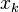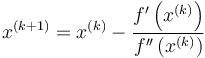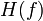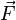Newton's method for optimization of a function of multiple variables

Definition

Suppose$f$ is a differentiable function of variables$x_1,x_2,\dots,x_n$. Newton's method for optimization is a method that aims to find points in the domain of$f$ where$f$ attains a local extremum.

Iterative step

Here, we describe how to get the next guess from a given guess.

In the case of a function$f$ of one variable$x$, the next guess from a given guess$x_k$ was given as:$x^{(k+1)} = x^{(k)} - \frac{f'\left(x^{(k)}\right)}{f''\left(x^{(k)}\right)}$

In our situation, the formula is as follows:$\vec{x}^{(k+1)} = \vec{x}^{(k)} - \left(H(f)\left(\vec{x}^{(k)}\right)\right)^{-1}(\nabla f)(\vec{x}^{(k)})$

Here,$H(f)$ is the Hessian matrix for$f$ (the analogue of the "second derivative") and is a$n \times n$ square matrix, while$\nabla f$ is the gradient vector for$f$ (the analogue of the "first derivative") and is a$.$-dimensional vector (expressed as a column vector).

Relation with Newton's method for root-finding for vector functions

How this method builds on that

We use the fact that point of local extremum implies critical point for a function of multiple variables to convert:

Problem of finding points of local extrema for the function$f$$\to$ Problem of finding the roots of the vector-valued function$\nabla f$ (which we then solve through Newton's method for root-finding for a vector-valued function of a vector variable)

However, it's worth noting that not all roots of the gradient vector are points of local extremum for$f$, and we need some analogue of derivative tests to figure out which ones are what. More on this below.

The dictionary is as follows:

• The vector-valued function$\vec{F}$ in the root-finding scenario equals the gradient vector$\nabla f$ in the optimization scenario.
• The Jacobian matrix$J(\vec{F})$ in the root-finding scenario equals the Hessian matrix$H(f)$ in the optimization scenario.

Symmetry of the matrix

Newton's method for optimization involves a somewhat narrower class of problems than Newton's method for root-finding. Explicitly, this is because, with Newton's method for root-finding, there are no constraints on how the Jacobian matrix at a given point could look (though there are the usual restrictions on how the Jacobian matrix as a matrix function can look). On the other hand, with Newton's method for root-finding, the Jacobian matrix of the gradient vector, which is now the Hessian, must be a symmetric matrix under appropriate differentiability assumptions (this follows from Clairaut's theorem on equality of mixed partials). Thus, some methods specifically for symmetric matrices may be applied here.

Second derivative test: Positive and negative definiteness of matrix

With Newton's method for optimization of a function of one variable, we used the second derivative test to detect whether the point we were approaching seems to be the correct type of extremum.

The analogous test here is the second derivative test for a function of multiple variables: it requires that the Hessian matrix be positive semidefinite (in the case of a local minimum) or negative semidefinite (in the case of a local maximum). If it's neither, we are probably quite far from a desirable root. Iterating the method might still prove helpful, but resetting to a random new value might also be helpful.